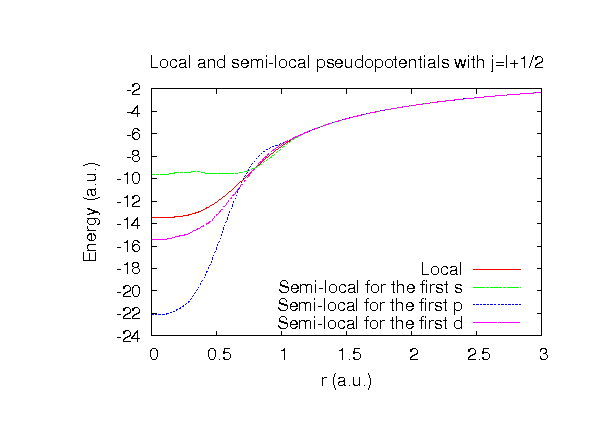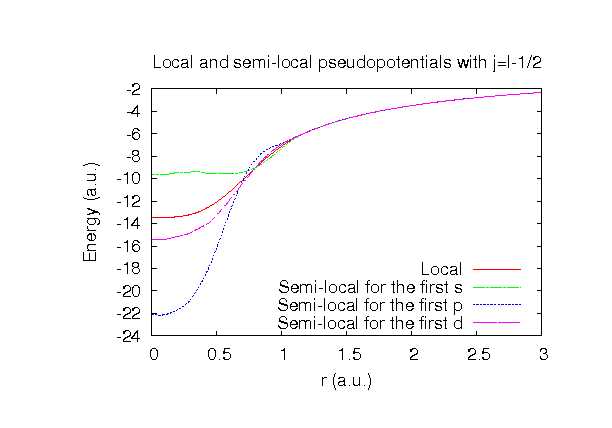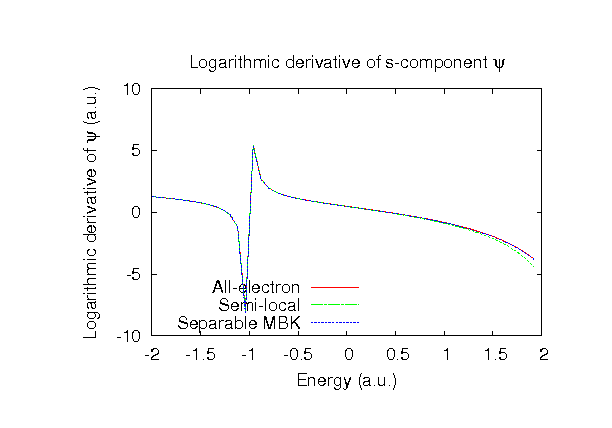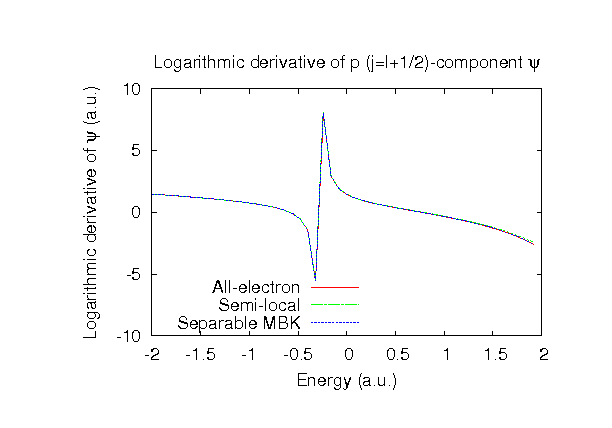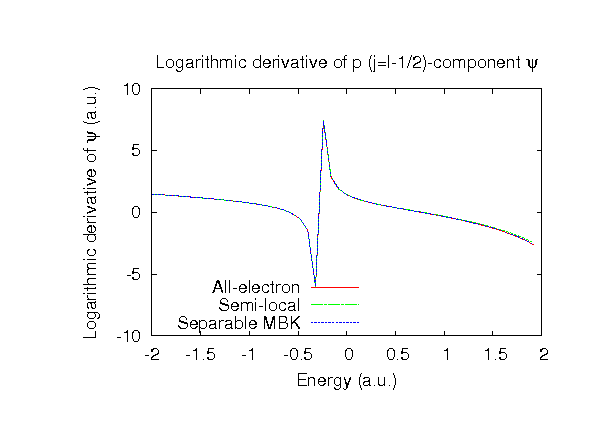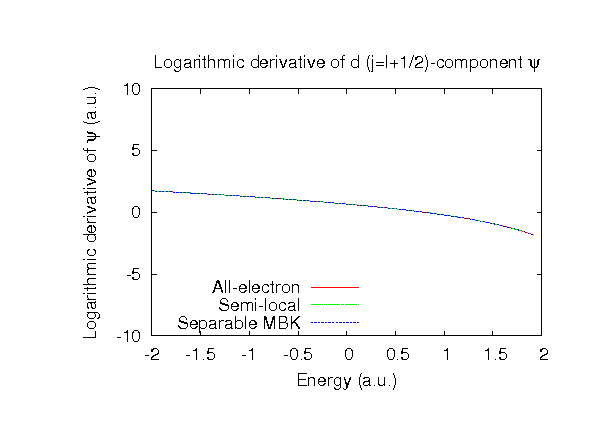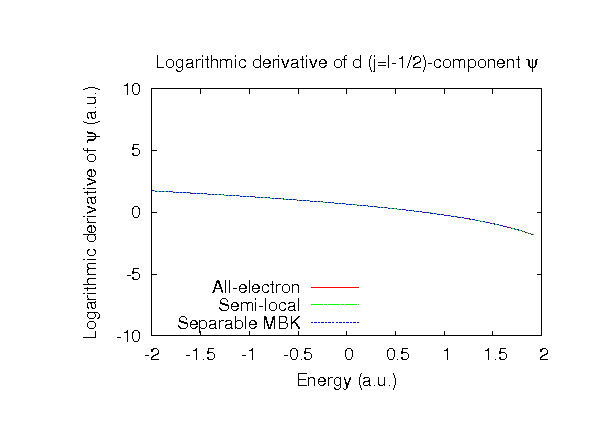### Fully relativistic pseudopotentials

Fully relativistic pseudopotentials generated by the MBK (PRB 47, 6728 (1993)) scheme within LDA (CA13) and GGA (PBE13) which contain a partial core correction and fully relativistic effects including spin-orbit coupling.

### Pseudo-atomic orbitals

The number below the symbol means a cutoff radius (a.u.) of the confinement potential. These file includes fifteen radial parts for each angular momentum quantum number l (=0,1,2,3). The basis functions were generated by variationally optimizing the corresponding primitive basis functions in the single atom, the dimer molecule, and the NaF bulk. The input files used for the orbital optimization can be found at F_opt.dat , F2_opt.dat , and NaF_opt.dat . Since F_CA13.vps and F_PBE13.vps include the 2s and 2p (7 electrons) as the valence states, the minimal basis set is F*.*-s1p1. Our recommendation for the choice of cutoff radius of basis functions is that F5.0.pao is enough for bulks, but F6.0.pao or F7.0.pao is preferable for molecular systems.

### Benchmark calculations by the PBE13 pseudopotential with the various basis functions

(1) Calculations of the total energy as a function of lattice constant of NaF in the rock salt structure, where the total energy is plotted relative to the minimum energy for each case. a0 and B0 are the equilibrium lattice constant and bulk modulus obtained by fitting to the Murnaghan equation of state. The difference between F6.0-s2p2d1 and F6.0-s3p3d2 in the total energy at the minimum point is 0.0044 eV/atom, where Na9.0-s3p2d1 was used as basis functions for Na for both the cases. An input file used for the OpenMX calculations can be found at NaF-EvsV.dat . For comparison the result by the Wien2k code is also shown, where the calculation was performed by default setting in the Ver. 10.1 of Wien2k except for the use of RMT x KMAX of 12.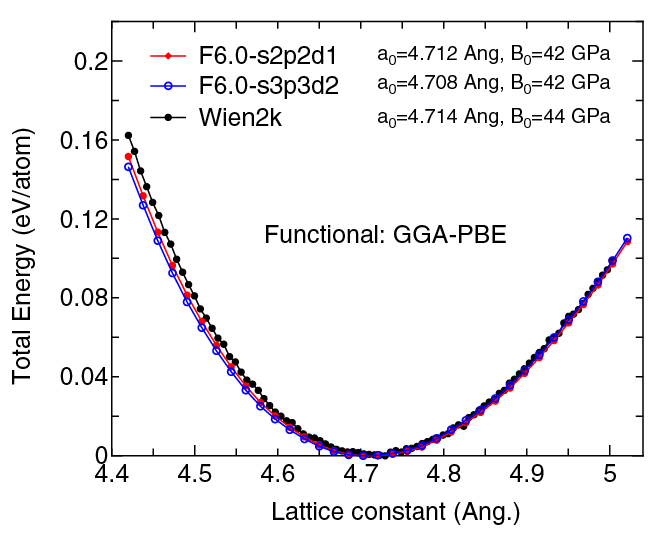(2) Calculations of the band dispersion of NaF in the rock salt structure, where the non-spin polarized collinear calculation with the lattice constant of 4.62 Ang. was performed using Na_PBE.vps, F_PBE13.vps, Na9.0-s3p2d1, and F6.0-s2p2d1, and the origin of the energy is taken to be the top of the valence band. The input file used for the OpenMX calculations can be found at NaF-Band.dat . For comparison the result by the Wien2k code is also shown, where the calculation was performed by default setting in the Ver. 10.1 of Wien2k except for the use of RMT x KMAX of 12.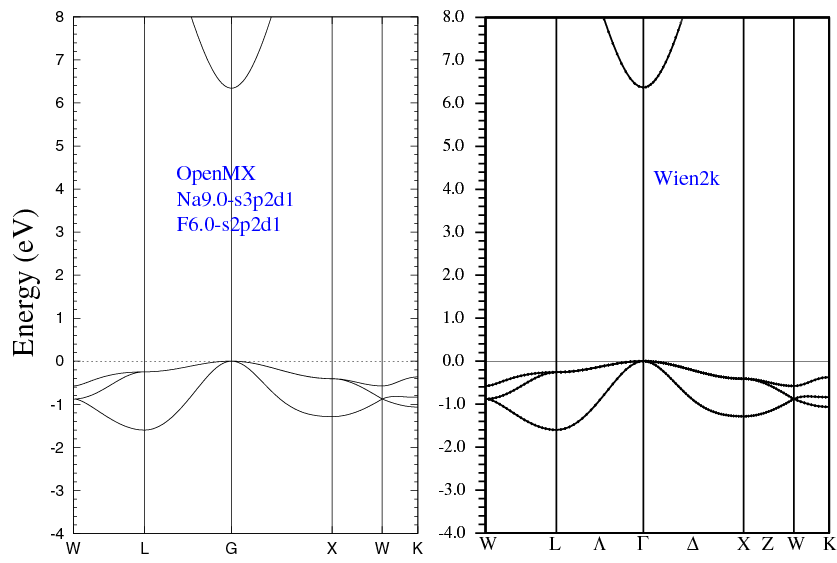(3) Calculations of the F2 molecule with S=1, where F_PBE13.vps and F7.0-s3p3d2 or F7.0-s4p4d4f3 were used. The input files used for the OpenMX calculations can be found at F2.dat , F.dat , and F_CP.dat .

 Equilibrium bond length (Ang.) Atomization energy (eV) Atomization energy (couterpoise corrected) (eV) F7.0-s3p3d2 1.455 2.406 2.399 F7.0-s4p4d4f3 1.432 2.615 2.602 Other calc. 1.430 a 2.236 a Expt. 1.412 b 1.6 b

a M.M. Odashima, K. Capelle, and S.B. Trickey, J. Chem. Theory Comput. 5, 798 (2009).
b Taken from Ref. (a)

### Supplementary information for the GGA (PBE13) pseudopotential